# Lesson Notes By Weeks and Term - Primary 2

The concept of 100

TERM: 1st Term

WEEK: 2

CLASS: Primary 2

AGE: 7 years

DURATION: 5 periods of 40 minutes each

DATE:

SUBJECT: Mathematics

TOPIC: The concept of 100

SPECIFIC OBJECTIVES: At the end of the lesson, the pupils should be able to

1. Read and write numbers that make up 100.
2. Sequence and compare numbers up to 100.
3. Order and compare whole numbers to 100.

INSTRUCTIONAL TECHNIQUES: Explanation, question and answer, demonstration, practical

INSTRUCTIONAL MATERIALS: 100 board per learner, 10 bottle tops per learner, Number symbol and name cards 21 to 50, ten frames, place value table per learner, Maths textbook

PERIOD 1: The concept of 100

 PRESENTATION TEACHER’S ACTIVITY PUPIL’S ACTIVITY STEP 1MENTAL MATHS The teacher begins the lesson with some mental calculationsWhich number comes after1 152 283 794 715 43 Which number comes before6 257 228 189 3110 50 Pupils respond and participate STEP 2CONCEPTDEVELOPMENT The teacher• Draws the following on the chalkboard.• Asks: How do you break 15 down into tens and ones? (15 is one ten and 5 ones or 10 + 5.)• Records this in the boxes below the15 (drawn on the board).• Completes the other 3 boxes on the board, asking different learners to come up to the board and discussing the solutions as they are done. (26 is 2 tens and 6 ones, 47 is 4 tensand 7 ones, 62 is 6 tens and 2 ones) CLASS ACTIVITYThe teacher• Gives each pair a 100 board and 10 bottle tops.• Revises counting forwards and backwards in 10s and 5s with 100 with the learners.• Using the bottle tops, asks the learners to put them on the numbers 91 to 100 one by one.• When the learners get to 99 asks them how many tens and ones in 99 ?( 9 tens and 9 ones)• Asks them what number will come next?• Discusses a 100 as ten groups of 10.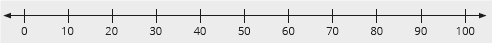ACTVITY IIThe teacher• Draws a number line in intervals of 10 to 100 on the board.• Asks the learners what can you tell me about this number line?• Discusses with them the intervals of ten.• Count forwards and backwards to 100 in 10s with the learners.• Using the number line asks learners questions such as: What is 10 more than 40? What is 10 less than 90?• Repeats using a variety of numbers.• Draws the number line below on the board in 5s from 0–100.• Asks the learners what can you tell me about this number line?• Discusses with them the intervals of five.• Counts forwards and backwards to 100 in 5s with the learners.• Using the number line, asks learners questions such as: What is 5 more than 55? What is 5 less than 95?• Repeats using a variety of numbers. Pupils pay attention and participate STEP 3CLASS-WORK 1 What is …?a The number that is 10 more than 40 _____b The number that is 10 less than 90 ____c The number that is 10 more than 70 ____d The number that is 10 less than 10 ____ 2 Complete the following patterns:3 Complete:a 10 + 40 = ___b 50 + 30 = ___c 20 + 70 = ___d 100 – 60 = ___e 30 – 10 = ___ Pupils attempt their class work STEP 4HOME-WORK Complete:a 30 + 70 = ___b 60 + 40 = ___c 10 + 80 = ___d 90 – 50 = ___e 100 – 20 = ___ The pupils writes it in their homework book STEP 5SUMMARY The teacher summarizes by reminding the pupils that in this lesson they learnt about 100. We also added and subtracted in 10s using our 100 boards and number lines to help us. She marks their class works, makes corrections where necessary and commends them positively

PERIOD 2: Comparing and sequencing numbers

PRESENTATION

TEACHER’S ACTIVITY

PUPIL’S ACTIVITY

STEP 1

MENTAL MATHS

The teacher begins the lesson with some mental calculations

Which number is between

1 7 and 9

2 12 and 14

3 18 and 20

4 25 and 27

5 19 and 21

6 34 and 36

7 39 and 41

8 26 and 24

9 31 and 29

10 43 and 45

Pupils respond and participate

STEP 2

CONCEPT

DEVELOPMENT

The teacher

• Sticks the number symbol and name cards for numbers 21 to 50 in a random order on

the board.

• Places the cards in the correct order while the learners read the number names.

• Asks the learners to look at numbers 21 to 50.

• Asks the learners if they notice anything special about these numbers.

What do they notice? (Possible answers may involve odd numbers/even numbers/tens and units.)

CLASS ACTIVITY

The teacher

Draws a 90 to 100 number line on the board.Calls on different learners to come to the board and fill in these numbers: 99, 91, 93, 97, 92, 94, 96, 95, 98.

When the number line labelling is complete, discusses the following questions with the learners:

- Which number is one less than 94? (93)

- One more than 94? (95)

- Two less than 94? (92)

- Two more than 94? (96)

Asks many such questions using different numbers in the range 90-100.

ACTIVITY II

The teacher

Draws 2 place value tables on the boards.

Pastes the number names 26 and 14 on the board above the place value tables.

Asks a learner to show 26 in the first table using base ten kits.

Asks another learner to show 14 in the second table using base ten kits.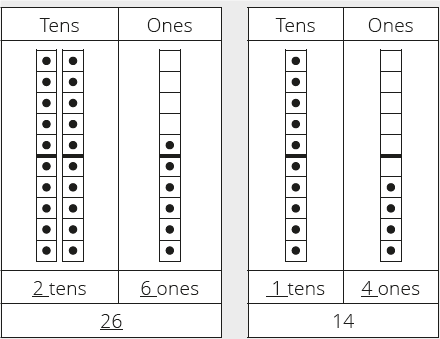Asks the learners the following questions:

- Which number is bigger? (26)

- How many more tens in 26 than in 14? (2 tens in 26 and 1 ten in 14, 1 more ten in 26)

- How many ones in 14?(4)

- How many more ones do I need to make 14 up to 20?(6)

- Discusses other pairs of numbers in a similar way.

Compares 57 and 32 (57 is bigger. 57 has 5 tens and 32 has 3 tens)

Compares 66 and 86 (86 is bigger. 86 has 8 tens and 66 has 6 tens)

Compares 56 and 26 (26 is smaller. 26 has 2 tens and 56 has 5 tens)

Compares 73 and 37 (37 is smaller. 37 has 3 tens and 73 has 7 tens)

Pupils pay attention and participate

STEP 3

CLASS-WORK

Note: Learners can use their base ten kits when they do this activity if they would like to.

1 Arrange these numbers from the smallest to the biggest: 30, 25, 29, 21.

2 Give two numbers that are bigger than 25.

3 Give two numbers that are smaller than 25.

4 Complete the pattern: 90, __, 92, 93, __, __, 96, __, 98, 99, __.

5 Circle the numbers that are smaller than 30 and bigger than 24.

 20 21 22 23 24 25 26 27 28 29 30

6 Circle the numbers that are smaller than 40 and bigger than 36.

 30 31 32 33 34 35 36 37 38 39 40

7 Write the number name for 32.

8 Write the number name for 48.

Pupils attempt their class work

STEP 4

HOME-WORK

1 Write the number name for 45.

2 Complete the following: 30 + ___ = 38.

3 Arrange these numbers from the biggest to the smallest: 93, 97, 95, 96, 94.

The pupils writes it in their homework book

STEP 5

SUMMARY

The teacher summarizes by reminding the pupils that in today’s lesson we found out that 100 comes after 99 when we are counting in 1s. We also worked with numbers between 0 and 99 to compare them to find the bigger/smaller number of a pair of numbers and to say how much bigger/smaller they are.

She marks their class works, makes corrections where necessary and commends them positively

PERIOD 3: Assessment

PRESENTATION

TEACHER’S ACTIVITY

PUPIL’S ACTIVITY

STEP 1

ORAL

ASSESSMENTS

The teacher asks questions on mental maths treated so far

Pupils respond and participate

STEP 2

DISCUSSION

The teacher discusses all the methods used by some learners in the oral assessments(some of the questions are solved on the board by the learners) and addresses any misconceptions that may have risen

Pupils pay attention and participate

STEP 3

WRITTEN ASSESSMENTS

1 Show the number 15 by filling in dots on the ten frames.

2 Write the number name for 100. ___________________________________

3 Write the number name for 41 ___________________________________

4 Circle the biggest number and make a cross over the smallest number.

 26 44 11 98 77 69 83

5. Arrange these numbers from biggest to smallest: 91, 100, 97, 90

6 Arrange these numbers from smallest to biggest: 81, 66, 72, 80

7 Write down three numbers that are bigger than 91, but smaller than 99.

8 Complete the following:

a 10 + 6 = _____

b 20 + 8 = _____

c 32 = 30 + ____

9 Complete the place value table.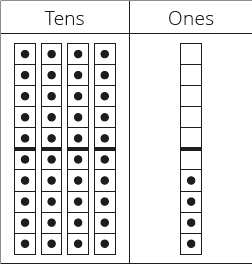Pupils attempt their class work

STEP 4

SUMMARY

The teacher marks the written assessments, corrects were necessary and commends the pupils

PERIOD 4: Comparing and ordering numbers

 PRESENTATION TEACHER’S ACTIVITY PUPIL’S ACTIVITY STEP 1MENTAL MATHS The teacher begins the lesson with some mental calculationsThe teachera Gives each learner a book. For example their maths textbook.b Repeats this exercise using pages 56, 77, 81 and 98.d Watches how the learners page through the books to get to page 40/41 etc. Do they count from 1 or do they skip a whole lot and slow down when they are near to thepage number that they are looking for?e Discusses how to page to a given page number (e.g. page 41) in the quickest way. Pupils respond and participate STEP 2CONCEPTDEVELOPMENT The teacher• Writes the following numbers on the board 63, 81, 57, 78, 95, 54, 72, 78, 90, 66, 89.• Asks the learners to put the number in order from smallest to biggest with you.• Discusses how to decide on the order. (look at the tens and units in the numbers.)• Asks the learners for the names of each of the numbers and write them next to each onefor example:63 – sixty three.  CLASS ACTIVITYThe teacher• Draws a 50 to 60 number line on the board.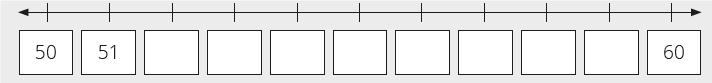• Calls on different learners to come to the board and fill in these numbers: 59, 53, 57, 52, 54, 56, 55, 58.• Asks the learners the following questions:- Which number is one less than 54? (53)- One more than 54? (55)- Two less than 54? (52)- Two more than 54? (56)• Asks many such questions using different numbers in the range 50-60. ACTIVITY IIThe teacher• Gives each learner a copy of a 100 board.• Asks the learners to count in tens from 10 to 100. They can refer to their boards if they want to.• Asks the learners to show you the following numbers on the number board: 44, 68, 70, 61, 86, 53, 99, 65 and 99.• Then asks them the following questions:- Which number is one less than 64? (63)- Which number is one more than 64? (65)- Which number is equal to 64? (64)• Allows learners to ask each other comparative questions, in the number range 0 to 100.- Which number is one ten less than 64? (54)- Which number is one ten more than 64? (74)- Which number is equal to 64? (64) Pupils pay attention and participate STEP 3CLASS-WORK 1 Write the number names for 65, 82, 49 and 98. 2 Fill in the missing numbers on the number line.a Circle all the numbers on the number line that are greater than 68.b Cross out all the numbers smaller than 65. 3 Complete the following:a 20 + ______ = 29b 40 + 4 = ______ 4 Fill in the missing numbers: 0, 10, __, __, __, 50, __, __, __, __, 100.  5 Write down the numbers 68 and 70. What is:a. the number that comes between them?b. the number that is 2 less than 68?c. the number that is 1 less than 70? Pupils attempt their class work STEP 4HOME-WORK 1 Complete the pattern: 68, 69, __, 71, __, __.2 50 + 6 = _____3 70 + 3 = _____4 What is two more than 71?5 What is two less than 71? The pupils writes it in their homework book STEP 5SUMMARY The teacher summarizes by reminding the pupils that in today’s lesson we used a number line to count and we used our 100 boards to find and discuss numbers up to 100. She marks their class works, makes corrections where necessary and commends them positively

PERIOD 5: Weekly Test/consolidations

TEACHER’S ACTIVITY: The teacher revises all the concepts treated from period 1-4 and gives the pupils follow through exercises, quiz and tests. She marks the exercises, makes corrections and commends the pupils positively.

PUPIL’S ACTIVITY: The pupils work on the worksheets and exercises give by the teacher individually

CONSOLIDATION

1 Fill in the missing multiples of 10:

a 10 , 20, 30, __, ___, 60, __, __, __, 100

b 100, 90, 80, __, __,__, __, __, __, 10.

c ___ , ____ , ____ , ____ , ____ , 50 , ____ , ____ , 20 , ____.

2 Complete the number lines.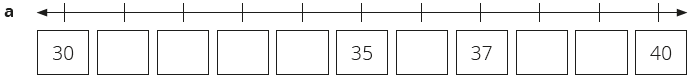3 Complete the place value table:4 20 + ___ = 27

5 ____ + 4 = 44

6 60 + 6 = ____

7 50 + _____ = 59

8 ____ + 2 = 82

9 ____ + 3 = 33